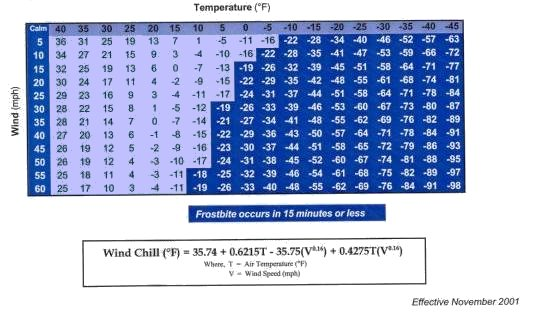Temperature Conversion Enter a number then click on the appropriate number to see the result. Fahrenheit ( o F ) Celsius ( o C )

Go to top

 Windchill Calculation Enter in the air temperature ( Tair ) in degrees Fahrenheit and wind speed in mph, then click on the Calculate WC to compute the windchill ( WC ). Tair mph

Go to top

 Relative Humidity Calculation Enter in the air temperature ( Tair ) and dew point temperature ( Tdp) in degrees Fahrenheit ( oF ) then click on the Calculate RH to compute the relative humidity ( RH ) Tair Tdp

Go to top

 Heat Index Calculation Enter in the air temperature ( Tair ) in degrees Fahrenheit and relative humidity ( RH ) in percent, then click on the Calculate HI to compute the heat index ( HI ). Tair RH

Go to top